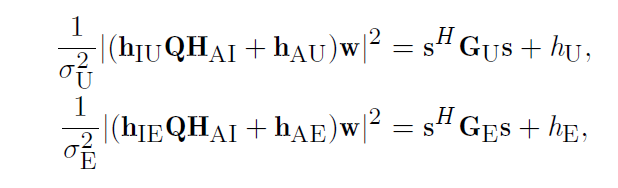# How to keep trace(IX) is real?

h1=conj(H)*conj(w2)*w2.’*H.’;
h2=conj(E)*conj(w1)*w1.’*E.’;
I=[diag(conj(A))*conj(D)*conj(w2)*w2.’*D.’*diag(A) diag(conj(A))*conj(D)*conj(w2)*w2.’*H.’
conj(H)*conj(w2)*w2.’*D.’*diag(A) 0];

cvx_begin
variable X(N+1,N+1) Hermitian
maximize (trace(I*X)+u*(h1+z1+h2))
subject to
trace(IX)+u(hA+z1)==1;
cvx_end

it shows up :
Expressions in objective functions must be real.

u*(hA+z1+hB) is real.
In my calculation, X=xx’, x is complex vector.A,D,H is complex matrix, and w1 is real vector. so trace(IX)=x’Ix.Then the trace(IX) is real.
i don’t know how to deal with the problem.please help me.Have you proven that the objective function must be real if there is no roundoff error? If so, use
`maximize(real(trace(I*X) +u*(h1+z1+h2))`
which will get rid of roundoff error level imaginary terms in `trace(I*X)`, which will cause an error if not eliminated. Or you could put `real(...)` around the whole objective function.

However, if it turns out that the objective function might not be real, even with no roundoff error, you might not be solving a problem which makes sense for your intended application.

If `u*(h1+z1+h2` is a constant, which it appears to be in your problem, it probably can serve no useful purpose to have it in the objective function, so why add any potential drama? If you want the optimal value of the objective function including that term, add it to the optimal objective value reported by CVX.

Thank you very much! u is also a variable. i forgot to write variable u.

I simulate this paper, by using the way above.it used SDR,my code is same to the above.
but when i simulate it ,the result X is a complex matrix, rather than a real one. I think there is something wrong. please help me.thanks!

The result of simulation shows u is real, it means u(h1+z1+h2)* is real. so it is my expected result.
I think maybe the way i defined the structure type of matrix X is wrong.

Have you worked out why problem (21) is equivalent to problem (19)?. I presume matters will be clarified once you do that.

Oh i see, if s is complex then S or X is a hermitian matrix. if s is real , then the matrix will be symmetric. if i want the result “X” to be a real matrix,then i need to change the word “hermitian” into" symmetric". I test it just now, the result is real, is that right?
if my assumption is right, there is an another question: I use the way above (i.e. maximize(real(trace(IX) +u(h1+z1+h2))),and let X is a hermitian one (i.e. it is complex). the result of trace(IX) +u(h1+z1+h2) is 1.9760e+03 + 4.3751e-14i, the imagine part is so small, and still exist. Should i assume that it is because of accuracy error? or there are still something wrong in my code.

if `abs(imag(expression)) < 1e-12`, for an `expression` which is theoretically supposed to be `real`,you can presume the imaginary part is due only o roundoff error,hence everything should be fine using r`eal(expression) `.

This is a commonly encountered “challenge” in CVX, for expressions such as `x'*H*x`, where `x` is complex and `H` is hemitian semidefinite. CVX is so fussy that imaginary terms of magnitude 1e-20 cause CVX to issue an error message, unless the real part is taken in order to eliminate the “nuisance” imaginary term.

As far as the correctness of what you did, I fer gain to my previous statement - the burden is on you to come up with a proper formulation in which you know which variables need to be complex, and which can be declared real.

Oh I see, Thank you very much for your guidance!